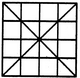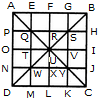# Non Verbal Reasoning - Analytical Reasoning - Discussion

Discussion Forum : Analytical Reasoning - Section 1 (Q.No. 17)
17.

Find the number of triangles in the given figure.36
40
44
48
Explanation:

The figure may be labelled as shown.The simplest triangles are APQ, AEQ, QTU, QRU, BGS, BHS, RSU, SUV, TUW, UWX, NWD, WDM, UVY, UXY, JCY and YKC i.e. 16 in number.

The triangles composed of two components each are QUW, QSU, SYU and UWY i.e. 4 in number.

The triangles composed of three components each are AOU, AFU, FBU, BIU, UIC, ULC, ULD and OUD i.e. 8 in number.

The triangles composed of four components each are QYW, QSW, QSY and SYW i.e. 4 in number.

The triangles composed of six components each are AUD, ABU, BUC and DUC i.e. 4 in number.

The triangles composed of seven components each are QMC, ANY, EBW, PSD, CQH, AGY, DSK and BJW i.e. 8 in number.

The triangles composed of twelve components each are ABD, ABC, BCD and ACD i.e. 4 in number.

Thus, there are 16 + 4 + 8 + 4 + 4 + 8 + 4 = 48 triangles in the figure.

Discussion:
6 comments Page 1 of 1.

Dilip Das said:   4 years ago
1. QSYW have 16 triangle.
2. APQE have 2 triangel (APQ and AEQ) like that all 4 corner of whole Square so: 2x4=8.
3. AOUF have 2 triangel (AOU and AFU) like that all 4 corner of whole Square so: 2x4=8.
4. ABCD have 16 triangle.

Total = 16+8+8+16 = 48 answer.

Bablu said:   4 years ago
What is the short trick?

Yousee said:   4 years ago
It is 44 triangle.